## 9.3.2 Deterministic Strategies

What constitutes a good solution to Formulation 9.7? Consider the game from the perspective of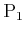. It seems reasonable to apply worst-case analysis when trying to account for the action that will be taken by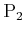. This results in a choice that is equivalent to assuming thatis nature acting under the nondeterministic model, as considered in Section 9.2.2. For a matrix game, this is computed by first determining the maximum cost over each row. Selecting the action that produces the minimum among these represents the lowest cost thatcan guarantee for itself. Let this selection be referred to as a security strategy for.

For the matrix game in (9.42), the security strategy is illustrated as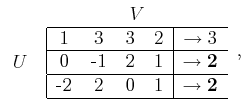(9.43)

in which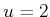and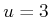are the best actions. Each yields a cost no worse than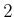, regardless of the action chosen by.

This can be formalized using the existing notation. A security strategy,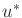, foris defined in general as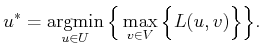(9.44)

There may be multiple security strategies that satisfy the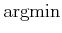; however, this does not cause trouble, as will be explained shortly. Let the resulting worst-case cost be denoted by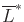, and let it be called the upper value of the game. This is defined as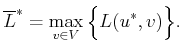(9.45)

Now swap roles, and consider the game from the perspective of, which would like to maximize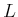. It can also use worst-case analysis, which means that it would like to select an action that guarantees a high cost, in spite of the action ofto potentially reduce it. A security strategy,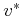, foris defined as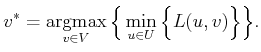(9.46)

Note the symmetry with respect to (9.44). There may be multiple security strategies for. A security strategyis just an upside-down'' version of the worst-case analysis applied in Section 9.2.2. The lower value,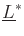, is defined as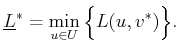(9.47)

Returning to the matrix game in (9.42), the last column is selected by applying (9.46):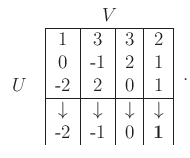(9.48)

An interesting relationship between the upper and lower values is that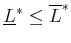for any game using Formulation 9.7. This is shown by observing that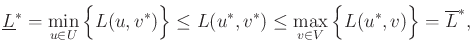(9.49)

in which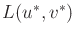is the cost received when the players apply their respective security strategies. If the game is played by rational DMs, then the resulting cost always lies betweenand.

Subsections
Steven M LaValle 2020-08-14